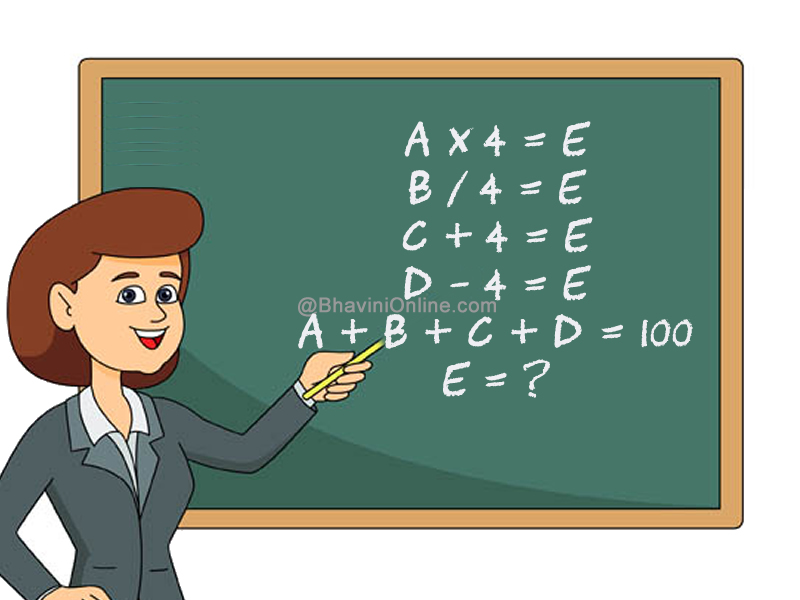# Fun Math Riddle: If A x 4 = E… Then, E = ?

You would need a pen & paper to solve this riddle.

Solve the given equations and find the value of E.

If;

A x 4 = E

B / 4 = E

C + 4 = E

D – 4 = E

A + B + C + D = 100

Then;

E = ?If you get the correct answer, share it with your friends on WhatsApp, Facebook and other social networking sites.

## One Reply to “Fun Math Riddle: If A x 4 = E… Then, E = ?”

1. Kalam says:

A×4 = E, so A = E/4
B/4 = E, so B = 4E
C+4 = E, so C = E-4
D-4 = E, so D = E+4
and
A+B+C+D=100.

now substitute the values that we got of A, B, C and D in the equation, to get,
(E/4) + 4E + E-4 + E+4 = 100
(E/4) + 4E + 2E = 100
(E/4) + 6E = 100
(E+24E)/4 = 100
E+24E = 100×4
25E = 400
E = 400/25
E = 16